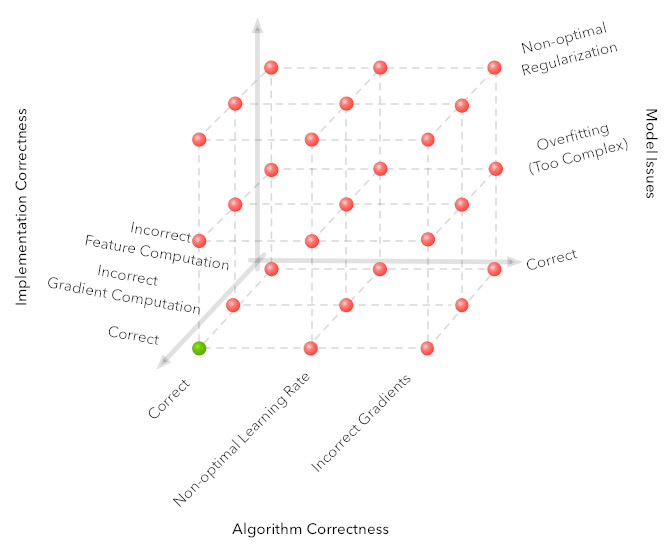# Debugging AI is a "hard problem"Writing code is a lot easier than fixing code! For a lot of well-understood reasons, code requires a lot of debugging to run safely and property, and different code structures and practices make debugging harder or easier. S. Zayd Enam, an AI researcher at Stanford, writes about the specific problems of debugging AI code, which is extremely difficult.

Specifically, debugging AI is exponentially harder than debugging other kinds of code; that's because AI has a lot more ways that it can go wrong than most other kinds of code, and to make things worse, it takes a long time to re-run training data through a machine-learning system after tweaking it, and until the run finishes, you won't know if your fix worked.

Traditional programming has two potential sources of mischief: problems with an algorithm, and problems with the code that implements the algorithm (think of cryptography, where a new vulnerability might occur because of a novel attack against a cipher, or because the programmer made a mistake programming the system the cipher is embedded in). The matrix of all the problems that can occur in this model is two dimensional: (all possible problems with the algorithm) * (all possible problems with the implementation).

But in AI, there two more dimensions get added to the problem space: the model and the data. Each one of these increases the list of possible bugs exponentially.

Our debugging process goes from a 2D grid to a 4D hypercube (three out of four dimensions drawn above for clarity). The fourth data dimension can be visualized as a sequence of these cubes (note that there is only one cube with a correct solution).

The reason this is 'exponentially' harder is because if there are n possible ways things could go wrong in one dimension there are n x n ways things could go wrong in 2D and n x n x n x n ways things can go wrong in 4D. It becomes essential to build an intuition for where something went wrong based on the signals available. Luckily for machine learning algorithms you also have more signals to figure out where things went wrong. For example, signals that are particularly useful are plots of your loss function on your training and test sets, actual output from your algorithm on your development data set and summary statistics of the intermediate computations in your algorithm.

Why is machine learning 'hard'? [S. Zayd Enam/Stanford]

(via Four Short Links)